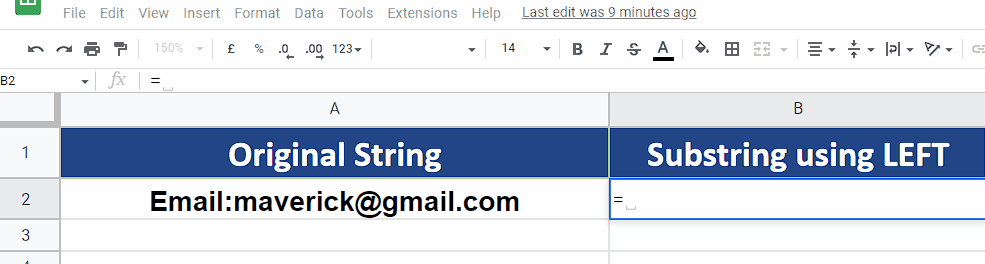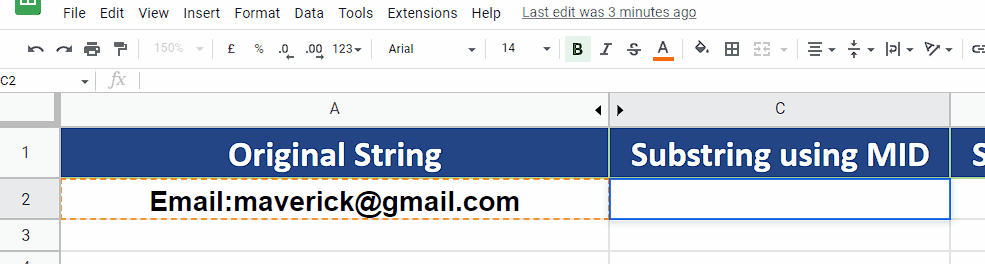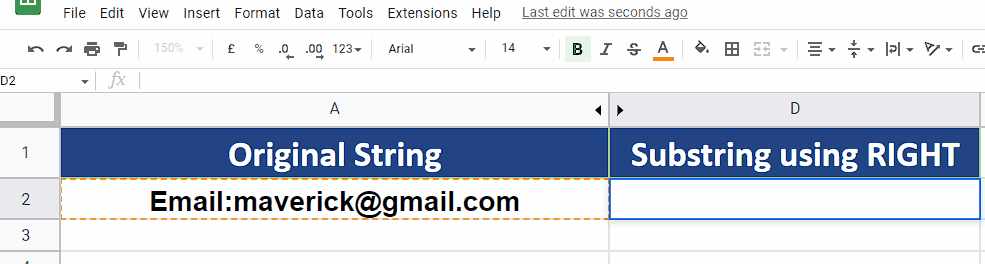# How to extract a substring from text in Google Sheet

Therefore, in today’s tutorial we are going to learn how to use some built-in text functions of Google Sheets to extract the required substrings. The following functions can be used to achieve the goal.

• LEFT to get the substring from beginning of the text
• MID to get the substring from middle of the text
• RIGHT to get the substring from end of the text

This tutorial covers only the basic methodology of using these functions, however, the usage of these functions is not limited to these examples only. The nature and use of these functions can be changed when used in conjunction with other functions, which is beyond the scope of this topic. Before jumping directly to the usage of these formulas, let’s understand the syntax of these formulas one by one.

## Syntax of the LEFT function

The syntax of this function is as follows;

LEFT(string, [number_of_characters])

The function takes two parameters:

• string:

This is a text string from which we want to extract the text.

• [number_of_characters]:

This parameter is optional. It specifies the number of characters that we wish to extract from the start of the string. For example, Maverick has 8 characters in total. If we wish to extract characters up to Mave then this argument should be 4. The default value is 1.

## Syntax of the MID function

The syntax of this function is as follows;

MID(string, starting_at, extract_length)

The function takes three parameters:

• string:

This is a text string from which we want to extract the text.

• starting_at:

This parameter specifies the number of characters from where we wish to start the extraction of character from the original string. For example, Maverick has 8 characters in total. If we wish to start extracting characters after Mave then this argument should be 5.

• extract_length:

This parameter specifies the number of characters to be extracted after the starting point, from the original string. For example, Maverick has 8 characters in total. If we wish to extract 4 characters after Mave then this argument should be 4.

## Syntax of the RIGHT function

The syntax of this function is as follows;

RIGHT(string, [number_of_characters])

The function takes two parameters:

• string:

This is a text string from which we want to extract the text.

• [number_of_characters]:

This parameter is optional. It specifies the number of characters that we wish to extract from the end of the string. For example, Maverick has 8 characters in total. If we wish to extract up to rick from the end of the string, then this argument should be 4. The default value is 1.

We have gone through the details of the syntax and the parameters of these formulas. Now we can learn how to use these formulas to extract any substring by following the steps mentioned below. Let’s assume that we have the text string as shown in the image above and we wish to extract a substring from it.Google Sheets has numerous functions and formulas to handle, manipulate and visualize data. In most of the cases we deal with numeric data, however, Google Sheets has a good library of functions and formulas to handle and manipulate the text data as well. Sometimes, we get raw text data as a result of web scraping or from other sources and we need to get the useful data from it. In such situations, we need to extract the useful substrings from the original text.

### Step 1 – Extract substring using LEFT function– We’ll use the following formula to extract the text Email from the original string. For this purpose, we’ll use the LEFT function in the following way;
=LEFT(A2, 5)
A2 contains the original string and 5 tells the formula to extract the first 5 characters from the start of the string as shown above.

### Step 2 – Extract substring using MID function– We’ll use the following formula to extract the text maverick from the original string. For this purpose, we’ll use the MID function in the following way;
=MID(A2, 7, 8)
A2 contains the original string. 7 tells the formula to start extraction from the 7th character from the start of the string i.e., m. 8 tells the formula that 8 characters have to be extracted starting from the 7th character from the start of the string.

### Step 3 – Extract substring using RIGHT function– We’ll use the following formula to extract the text gmail.com from the original string. For this purpose, we’ll use the RIGHT function in the following way;
=RIGHT(A2, 9)
A2 contains the original string and 9 tells the formula to extract 9 characters from the end of the string as shown above.